Seuls les membres ayant 30 points peuvent parler sur le chat.

## Forum Casio - Projets de programmation

Index du Forum » Projets de programmation » Mandelbrot Generator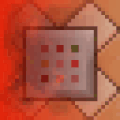Redcmd Hors ligne Membre Points: 273 Défis: 5 Message

#### Mandelbrot Generator

Posté le 15/06/2019 10:38

Hello allô

Default 1x zoom takes 7sec
Max zoom takes around 5-10min
It has a max zoom of 2^50: over one Quadrillion!
Going over 2^48 can be rather buggy
This is because numbers are limited to the 8 byte double variables

Attached file is both SH4 and SH3 compatible: MANDEL.G1A

This does need the 'MonochromeLib' libs the code comes with it now

Controls
[-] Zoom out
[+] Zoom in
[F1] Hide/show HUD which contains Cords, Zoom level and Max Iterations. (Heads Up Display)
[F2] Changes colours of camera rectangle: Black, White & Inverted
[AC] Resets screen back to default state
[EXE] Draw set
[EXIT] Stop drawing the Mandelbrot (If it's taking too long)
[REPLAY] Move camera rectangle around (Arrow Keys: [LEFT], [RIGHT], [UP], [DOWN])

How can I optimize this code to run faster or zoom in further?

#include "fxlib.h"
#include "stdio.h"

#define TRUE 1
#define FALSE 0

typedef enum { ML_TRANSPARENT = -1, ML_WHITE, ML_BLACK, ML_XOR, ML_CHECKER } ML_Color;
typedef char* (*sc_cpv)(void);
const unsigned int sc0135[] = { 0xD201D002, 0x422B0009, 0x80010070, 0x0135 };

unsigned int key;                //pause until key press
int kcode1, kcode2;                //row & col keycode for Bkey_GetKeyWait()
char unused;                    //unused (cause CASIO dumb dumb)
unsigned short dispX, dispY;    //cords on display when drawing mandelbrot

void ML_clear_vram() {
int i, end, * pointer_long, vram;
char* pointer_byte;
end = 4 - vram & 3;
pointer_byte = (char*)vram;
for (i = 0; i < end; i++) pointer_byte[i] = 0;
pointer_long = (int*)(vram + end);
for (i = 0; i < 255; i++) pointer_long[i] = 0;
pointer_byte += 1020 + end;
end = vram & 3;
for (i = 0; i < end; i++) pointer_byte[i] = 0;
}

void ML_display_vram() {
char* LCD_register_selector = (char*)0xB4000000, * LCD_data_register = (char*)0xB4010000, * vram;
int i, j;
for (i = 0; i < 64; i++) {
*LCD_register_selector = 4;
*LCD_data_register = i | 192;
*LCD_register_selector = 4;
*LCD_data_register = 0;
*LCD_register_selector = 7;
for (j = 0; j < 16; j++)
*LCD_data_register = *vram++;
}
}

void ML_display_vram_row(int row) {            //faster than ML_display_vram() which displays the entire screen instead of a single row
unsigned char i;
char* LCD_register_selector = (char*)0xB4000000, *LCD_data_register = (char*)0xB4010000, *vram;
vram = (row << 4) + ML_vram_adress();
*LCD_register_selector = 4;
*LCD_data_register = row | 192;
*LCD_register_selector = 4;
*LCD_data_register = 0;
*LCD_register_selector = 7;
for (i = 0; i < 16; i++)
* LCD_data_register = *vram++;
}

void ML_horizontal_line(int y, int x1, int x2, ML_Color color) {
int i;
char checker;
if (y & ~63 || (x1 < 0 && x2 < 0) || (x1 > 127 && x2 > 127))
return;
if (x1 > x2) {
i = x1;
x1 = x2;
x2 = i;
}
if (x1 < 0)
x1 = 0;
if (x2 > 127)
x2 = 127;
switch (color) {
case ML_BLACK:
if (x1 >> 3 != x2 >> 3) {
vram[(y << 4) + (x1 >> 3)] |= 255 >> (x1 & 7);
vram[(y << 4) + (x2 >> 3)] |= 255 << 7 - (x2 & 7);
for (i = (x1 >> 3) + 1; i < x2 >> 3; i++)
vram[(y << 4) + i] = 255;
} else
vram[(y << 4) + (x1 >> 3)] |= (255 >> (x1 % 8 + 7 - x2 % 8)) << (7 - (x2 & 7));
break;
case ML_WHITE:
if (x1 >> 3 != x2 >> 3) {
vram[(y << 4) + (x1 >> 3)] &= 255 << 8 - (x1 & 7);
vram[(y << 4) + (x2 >> 3)] &= 255 >> 1 + (x2 & 7);
for (i = (x1 >> 3) + 1; i < x2 >> 3; i++)
vram[(y << 4) + i] = 0;
} else
vram[(y << 4) + (x1 >> 3)] &= (255 << 8 - (x1 & 7)) | (255 >> 1 + (x2 & 7));
break;
case ML_XOR:
if (x1 >> 3 != x2 >> 3) {
vram[(y << 4) + (x1 >> 3)] ^= 255 >> (x1 & 7);
vram[(y << 4) + (x2 >> 3)] ^= 255 << 7 - (x2 & 7);
for (i = (x1 >> 3) + 1; i < (x2 >> 3); i++)
vram[(y << 4) + i] ^= 255;
} else
vram[(y << 4) + (x1 >> 3)] ^= (255 >> ((x1 & 7) + 7 - (x2 & 7))) << (7 - (x2 & 7));
break;
case ML_CHECKER:
checker = (y & 1 ? 85 : 170);
if (x1 >> 3 != x2 >> 3) {
vram[(y << 4) + (x1 >> 3)] &= 255 << 8 - (x1 & 7);
vram[(y << 4) + (x2 >> 3)] &= 255 >> 1 + (x2 & 7);
vram[(y << 4) + (x1 >> 3)] |= checker & 255 >> (x1 & 7);
vram[(y << 4) + (x2 >> 3)] |= checker & 255 << 7 - (x2 & 7);
for (i = (x1 >> 3) + 1; i < x2 >> 3; i++)
vram[(y << 4) + i] = checker;
} else {
vram[(y << 4) + (x1 >> 3)] &= (255 << 8 - (x1 & 7)) | (255 >> 1 + (x2 & 7));
vram[(y << 4) + (x1 >> 3)] |= checker & (255 >> (x1 % 8 + 7 - x2 % 8)) << (7 - (x2 & 7));
}
break;
}
}

void ML_vertical_line(int x, int y1, int y2, ML_Color color) {
int i, j;
char checker, byte, * vram = ML_vram_adress();
if (x & ~127 || (y1 < 0 && y2 < 0) || (y1 > 63 && y2 > 63)) return;
if (y1 > y2) {
int tmp = y1;
y1 = y2;
y2 = tmp;
}
if (y1 < 0) y1 = 0;
if (y2 > 63) y2 = 63;

i = (y1 << 4) + (x >> 3);
j = (y2 << 4) + (x >> 3);
switch (color) {
case ML_BLACK:
byte = 128 >> (x & 7);
for (; i <= j; i += 16)
vram[i] |= byte;
break;
case ML_WHITE:
byte = ~(128 >> (x & 7));
for (; i <= j; i += 16)
vram[i] &= byte;
break;
case ML_XOR:
byte = 128 >> (x & 7);
for (; i <= j; i += 16)
vram[i] ^= byte;
break;
case ML_CHECKER:
byte = 128 >> (x & 7);
checker = y1 & 1 ^ x & 1;
for (; i <= j; i += 16) {
if (checker) vram[i] &= ~byte;
else vram[i] |= byte;
checker = !checker;
}
break;
}
}

void ML_pixel(int x, int y, ML_Color color) {
if (x & ~127 || y & ~63) return;
switch (color) {
case ML_BLACK:
vram[(y << 4) + (x >> 3)] |= 128 >> (x & 7);
break;
case ML_WHITE:
vram[(y << 4) + (x >> 3)] &= ~(128 >> (x & 7));
break;
case ML_XOR:
vram[(y << 4) + (x >> 3)] ^= 128 >> (x & 7);
break;
case ML_CHECKER:
if (y & 1 ^ x & 1) vram[(y << 4) + (x >> 3)] &= ~(128 >> (x & 7));
else vram[(y << 4) + (x >> 3)] |= 128 >> (x & 7);
break;
}
}

double divByPow(double n, double x, int p) {        //Divide OR Times n by x, p times (n / x^p): used for numbers bigger than 2^32 (int limit)
if (p < 0)
for (; p < 0; p++)
n *= x;
else
for (; p > 0; p--)
n /= x;
return n;
}

void stop(void) {            //stops drawing set if user presses [EXIT] or [MENU]
if (Bkey_GetKeyWait(&kcode1, &kcode2, 1, 0, 1, &unused))
if (kcode1 == 4 && (kcode2 == 8 || kcode2 == 9)) {
dispX = 128;    //Very hacky stop function
dispY = 64;
}
}

int AddIn_main(int isAppli, unsigned short OptionNum) {        //Main function
unsigned int graphZoom = 1;                //zoom level for graph
char screenZoom;                        //zoom level on screen (rectangle)
int screenX1, screenX2;                    //corner X cords for drawing rectangle to screen
int screenY1, screenY2;                    //corner Y cords for drawing rectangle to screen
unsigned char string;                //Used in converting int/double to char
char HUD = TRUE;                        //Heads Up Display: Cords, Zoom level & Max iteration: toggle with [F1]
char colour = ML_XOR;                    //Colour of rectangle: Black, White or Inverted
int screenX, screenY;                    //offset cords on screen from 0,0 for rectangle
double graphX = 0, graphY = 0;            //cords on graph - where to center mandelbrot
double graphMove;                        //amount graphX & Y changes by when moving rectangle around
int screenMove;                            //amount screenX & Y changes by when moving rectangle around with arrow keys
short tempPixel = 0;                    //Write pixels to temp variable then write the entire 2bytes to VRAM all at once

register double zr, zi;                    //zr is real, zi imaginary
register double zr2, zi2;                //zr2 = zr^2, zi2 = zi^2
register double x1 = -2.0;                //bounding box cords on graph
register double x2 = 2.0;                //bounding box cords on graph
register double y1 = -1.0;                //bounding box cords on graph
register double y2 = 1.0;                //bounding box cords on graph
register double x, y;                    //pixel cords on graph tested if in set
register double xIsz, yIsz;                //amount x/y increases by when ploting graph
register unsigned short iMax = 32;        //max iterations
register unsigned short i;                //iterations

while (TRUE) {

SetTimer(1, 200, stop);
ML_clear_vram();
ML_display_vram();

xIsz = (x2 - x1) / 128;
yIsz = (y2 - y1) / 64;

y = y1;
for (dispY = 0; dispY < 64; dispY++) {
x = x1;
y += yIsz;
for (dispX = 0; dispX < 128; dispX++) {
zr = x;
zi = y;
for (i = 0; i < iMax; i++) {
zr2 = zr * zr;
zi2 = zi * zi;
if (zr2 + zi2 > 4)
break;
zi = zr * zi;
zi += zi + y;
zr = zr2 - zi2 + x;
}
tempPixel = (tempPixel << 1) | (i == iMax);
if ((dispX & 7) == 7)
*vram++ = tempPixel;
x += xIsz;
}
ML_display_vram_row(dispY);
}
SaveDisp(1);
KillTimer(1);
screenX = 0;
screenY = 0;
screenZoom = 1;
Bkey_GetKeyWait(&kcode1, &kcode2, 2, 1, 1, &unused);
do {
GetKey(&key);
screenMove = screenZoom > 4 ? 1 : divByPow(16, 2, screenZoom);
graphMove = screenZoom > 4 ? divByPow(1, 2, graphZoom - (double)screenZoom) : divByPow(16, 2, graphZoom);
switch (key) {
case KEY_CHAR_PLUS:
if (graphZoom < 51) {
graphZoom++;
screenZoom++;
}
break;
case KEY_CHAR_MINUS:
if (graphZoom) {
graphZoom--;
screenZoom--;
}
break;
case KEY_CTRL_UP:
screenY -= screenMove;
graphY -= graphMove;
break;
case KEY_CTRL_DOWN:
screenY += screenMove;
graphY += graphMove;
break;
case KEY_CTRL_LEFT:
screenX -= screenMove;
graphX -= graphMove;
break;
case KEY_CTRL_RIGHT:
screenX += screenMove;
graphX += graphMove;
break;
case KEY_CTRL_F1:
HUD = !HUD;
break;
case KEY_CTRL_F2:
if (colour)
colour--;
else
colour = ML_XOR;
break;
case KEY_CTRL_F3:
//Gray scale, by refreshing screen multiple times per sec at different max iterations (iMax)
break;
case KEY_CTRL_AC:
graphZoom = 1;
graphX = 0;
graphY = 0;
screenZoom = 1;
screenX = 0;
screenY = 0;
key = KEY_CTRL_EXE;
break;
}
RestoreDisp(1);
iMax = 8 * (graphZoom + 3);

if (screenZoom < 8) {
screenX1 = 65 - divByPow(128, 2, screenZoom) + screenX;
screenX2 = 62 + divByPow(128, 2, screenZoom) + screenX;
screenY1 = 32 - (screenZoom > 6 ? 1 : divByPow(64, 2, screenZoom)) + screenY;
screenY2 = 31 + (screenZoom > 6 ? 0 : divByPow(64, 2, screenZoom)) + screenY;
ML_horizontal_line(screenY1, screenX1, screenX2, colour);
ML_horizontal_line(screenY2, screenX1, screenX2, colour);
ML_vertical_line(screenX1 - 1, screenY1, screenY2, colour);
ML_vertical_line(screenX2 + 1, screenY1, screenY2, colour);
} else
ML_pixel(screenX + 64, screenY + 31, colour);

x1 = divByPow(-4, 2, graphZoom) + (0.03125 * graphX);
x2 = divByPow(4, 2, graphZoom) + (0.03125 * graphX);
y1 = divByPow(-2, 2, graphZoom) + (0.03125 * graphY);
y2 = divByPow(2, 2, graphZoom) + (0.03125 * graphY);

if (HUD == TRUE) {
sprintf(&string, "X1:%f", x1);
PrintMini(0, 0, string, 0);
sprintf(&string, "Y1:%f", y1);
PrintMini(0, 6, string, 0);
sprintf(&string, "X2:%f", x2);
PrintMini(81, 53, string, 0);
sprintf(&string, "Y2:%f", y2);
PrintMini(81, 59, string, 0);
sprintf(&string, "MaxI:%u", iMax);
PrintMini(0, 53, string, 0);
if (graphZoom > 32)
sprintf(&string, "Zoom:2^%ux", graphZoom - 1);
else
sprintf(&string, "Zoom:%ux", (int)divByPow(1, 2, -graphZoom + 1));
PrintMini(0, 59, string, 0);
}

ML_display_vram();

} while (key != KEY_CTRL_EXE);
}
return 0;
}

#pragma section _BR_Size
unsigned long BR_Size;
#pragma section
#pragma section _TOP
int InitializeSystem(int isAppli, unsigned short OptionNum) {
}
#pragma section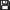Fichier joint

Précédente 1, 2, 3, 4, 5, 6, 7, 8 SuivanteRedcmd Hors ligne Membre Points: 273 Défis: 5 Message

Citer : Posté le 27/06/2019 01:26 | #

Maybe he's only using floats, not doubles
Only calcuating one half of the set and just flipping the image (because top and bottom of the set is the same)
tho, even when rendering just the top half of the set his is still faster
maybe he is using fixed point?
he is only displaying the pic after the set is calculated, but I tried that too, but that didn't change much
by default the mex iterations is set at 50 on his and 32 on my
RedCMD#4299 - Discord
Mandelbrot SNKEmini Minesweeper SudokuLephenixnoir Hors ligne Administrateur Points: 18125 Défis: 142 Message

Citer : Posté le 27/06/2019 01:54 | #

Using floats would be an idea, but I think you tried that too, am I right?

Fixed point is still a possibility. You could try with 32 bits and later port it to 64-bits if the performance is acceptable and you want more precision. The cost would be changing compiler.

To be honest I'm curious about how fast it can get, I'm just upset we could miss a simple optimization opportunity.Redcmd Hors ligne Membre Points: 273 Défis: 5 Message

Citer : Posté le 27/06/2019 03:31 | #

Yea I tried floats
it did help a bit tho not as much as we are looking for
only doubles are 32bits, ints are 16bits
I would still able to test my programs in the emulator on the SDK, if I compile with GCC?
RedCMD#4299 - Discord
Mandelbrot SNKEmini Minesweeper SudokuLephenixnoir Hors ligne Administrateur Points: 18125 Défis: 142 Message

Citer : Posté le 27/06/2019 04:30 | #

only doubles are 32bits, ints are 16bits

Doesn't seem right to me, doubles are always 64 bits otherwise they're called floats, because that's what they are. On this machine ints also happen to be 32 bits.

I would still able to test my programs in the emulator on the SDK, if I compile with GCC?

Yes, it is supposed to work. In the past we had trouble because the program generating the g1a header missed a field. Recent g1a wrappers have this fixed and it's been seen to work. I've never used it myself because I haven't used the SDK in ages but yeah basically it works.Redcmd Hors ligne Membre Points: 273 Défis: 5 Message

Citer : Posté le 07/11/2019 06:34 | #

Turns out he used fixed point precision
I did the same and its sooo much faster nowBut now I can only zoom into about 1024x, if I go futher it starts breaking down
6:26 was the best

How would I go about getting 64bit variables to work?
Is it possible to get GCC to work on Windows
or would I have to edit the code in assembler

How does the calculator support 64bit ints?
is it a 8bit processor that loops 8 times?

oh yea and doubles are 64bits, floats 32bits, ints 32bits, shorts 16bits, chars 16 8bits
typing long infront doesn't do anything
RedCMD#4299 - Discord
Mandelbrot SNKEmini Minesweeper SudokuLephenixnoir Hors ligne Administrateur Points: 18125 Défis: 142 Message

Citer : Posté le 07/11/2019 09:03 | #

Ah, my intuition wasn't wrong. Glad to see you closed the gap!So, for 64-bit variables you can either use GCC which has support for int64_t and even fixed-point primitives.

Or you can use assembler for the most critical part, which isn't too difficult. You add in two instructions with addc then add, and multiply using muls.l which multiplies 32×32→64-bit several times. The processor is 32-bit so that would be "twice the work" (simplifying a lot) instead of "8 times the work".oh yea and doubles are 64bits, floats 32bits, ints 32bits, shorts 16bits, chars 16bits
typing long infront doesn't do anything

Yes longs are still 32 bit. char is 8-bit though.Redcmd Hors ligne Membre Points: 273 Défis: 5 Message

Citer : Posté le 07/11/2019 09:18 | #

How to install GCC on windows (fxSDK?)
or what assembler editor do you recommend
RedCMD#4299 - Discord
Mandelbrot SNKEmini Minesweeper SudokuLephenixnoir Hors ligne Administrateur Points: 18125 Défis: 142 Message

Citer : Posté le 07/11/2019 09:26 | #

To have GCC on Windows, you can't use the fxSDK directly because it's not compatible, but you can follow the GCC tutorial provided you have a Unix-like environment such as WSL on Windows 10, or Cygwin.

Or you can look for pre-built binaries. I don't have links right now but I know some exist with the PrizmSDK or Intelligide's Prizm SDK. He made a adaptation of the GCC tutorial but this uses minGW so... might not be the correct target depending on your preferences.

-

You can edit assembler code in .src files directly in the SDK.Redcmd Hors ligne Membre Points: 273 Défis: 5 Message

Citer : Posté le 07/11/2019 20:07 | #

What .src files?
I found Mandel.lst in the debug folder
its filled with assembler code, but if I modify it, when the SDK rebuilds the program, wont it overwrite it?
RedCMD#4299 - Discord
Mandelbrot SNKEmini Minesweeper SudokuLephenixnoir Hors ligne Administrateur Points: 18125 Défis: 142 Message

Citer : Posté le 07/11/2019 20:11 | #

No, I mean you can create .src files of your own, just as you create .c files, to write assembly code. Then you should add them to the project and build as usual.Redcmd Hors ligne Membre Points: 273 Défis: 5 Message

Citer : Posté le 07/11/2019 22:12 | #

Im looking at C.Basic source code
theres a file called fx_syscall.src
which contains:
.EXPORT _Cursor_SetFlashMode
_Cursor_SetFlashMode:
mov.l SYSCALL,r1
mov.l #H'13A,r0
jmp @r1
nop

in the .c files I found
Cursor_SetFlashMode(0);

The pass in variable comes from: mov.l SYSCALL,r1 right?
mov.l #H'13A,r0 is the main command(s) being run
jmp @r1 takes it back to the code it was at
nop why does this need to be run? does the cpu need to wait a cycle for memory to load correctly?

is there some tutorials or examples on assembler I can look at?
whats the list of instructions
what does each of the registers hold. is there any special ones?
RedCMD#4299 - Discord
Mandelbrot SNKEmini Minesweeper SudokuLephenixnoir Hors ligne Administrateur Points: 18125 Défis: 142 Message

Citer : Posté le 07/11/2019 22:19 | #

The general-purpose registers are r0..r7 (caller-saved) and r8..r15 (callee-saved), r15 is the stack pointer.

Arguments go to r4..r7 then on the stack. So here the cursor flash mode is in r4. What this function does is jump to the syscall table with the syscall number in r0 (this is an unconvential place for a parameter to be). All the arguments are implictly forwarded because r4..r7 and the stack are not changed.

The SuperH has no branch prediction, thus they designed "delay slots" to avoid losing pipeline time when executing jumps. Basically, the instruction after the jump is executed before the jump occurs, filling an empty slot in the pipeline. Not all instructions can be in a delay slot. Also, values that are updated in the delay slot will not change the target of the jump. Here we could load r0 in the delay slot, but not r1 for instance.

When calling a function such as Cursor_SetFlashMode(), the return-to address is placed in register pr automatically by the call instruction. Here we jump to the syscall table with a regular jump that does not update pr. The syscall executes, and when it calls the rts instruction to return from function, it will immediately go back to the pr address, which is the caller of Cursor_SetFlashMode(). You may know this trick, which is called tail call optimization.

Ajouté le 07/11/2019 à 22:20 :
(Oops, sent the message by accident, sorry.)

You will definitely need some tutorial and documentation if you want to get this right. You can start with Ziqumu's tutorial, just don't bother with the manual disassembling in the SDK: https://www.planet-casio.com/Fr/programmation/tutoriels.php?id=43

Here is the CPU manual: https://bible.planet-casio.com/common/hardware/mpu/sh3_manual.pdfRedcmd Hors ligne Membre Points: 273 Défis: 5 Message

Citer : Posté le 08/11/2019 07:50 | # |Fichier joint

Im trying to mess around with it, tho I cant seem to get a blank test .src file working
and once I've got it working (in the future), how do I know which registers to use?
like if the rest of the program is using r3, and I go and use that, will it break?
or will the compiler see Im using and not use that one
or will I have to push all registers to the stack

r0-r7 is fine to use I guess

Thanks for those linksI've shrunk the code down and moved it all to one file (just for testing)
mandel.c
#include "stdio.h"

typedef char* (*sc_cpv)(void);
const unsigned int sc0135[] = { 0xD201D002, 0x422B0009, 0x80010070, 0x0135 };

void ML_clear_vram() {
int i, end, * pointer_long, vram;
char* pointer_byte;
end = 4 - vram & 3;
pointer_byte = (char*)vram;
for (i = 0; i < end; i++) pointer_byte[i] = 0;
pointer_long = (int*)(vram + end);
for (i = 0; i < 255; i++) pointer_long[i] = 0;
pointer_byte += 1020 + end;
end = vram & 3;
for (i = 0; i < end; i++) pointer_byte[i] = 0;
}

void ML_display_vram() {
char* LCD_register_selector = (char*)0xB4000000, * LCD_data_register = (char*)0xB4010000, * vram;
int i, j;
for (i = 0; i < 64; i++) {
*LCD_register_selector = 4;
*LCD_data_register = i | 192;
*LCD_register_selector = 4;
*LCD_data_register = 0;
*LCD_register_selector = 7;
for (j = 0; j < 16; j++)
*LCD_data_register = *vram++;
}
}

void ML_display_vram_row(int row) {
unsigned char i;
char* LCD_register_selector = (char*)0xB4000000, * LCD_data_register = (char*)0xB4010000, * vram;
vram = (row << 4) + ML_vram_adress();
*LCD_register_selector = 4;
*LCD_data_register = row | 192;
*LCD_register_selector = 4;
*LCD_data_register = 0;
*LCD_register_selector = 7;
for (i = 0; i < 16; i++)
*LCD_data_register = *vram++;
}

int AddIn_main(int isAppli, unsigned short OptionNum) {
unsigned int graphZoom = 1;
register int zr, zi;
register int zr2, zi2;
register int x, y;
register int Isz;
register int iMax;
register int xStart, yStart;
register unsigned short i;
register unsigned short col, row;
register short tempPixel = 0;

unsigned char string;

foo();

ML_clear_vram();

while (1) {

iMax = 8 * graphZoom + 24;

sprintf(&string, "%d", iMax);
PrintXY(30, 56, string, 0);

ML_display_vram();

Isz = 0x400000 >> graphZoom;
xStart = 0x1000000 - (0x10000000 >> graphZoom);
yStart = -0x8000000 >> graphZoom;

y = yStart;
for (row = 0; row < 64; row++) {
x = xStart;
y += Isz;
for (col = 0; col < 128; col++) {
zr = x;
zi = y;
for (i = 0; i < iMax; i++) {
zr >>= 13;
zi >>= 13;
zr2 = zr * zr;
zi2 = zi * zi;
if (zr2 + zi2 > 0x10000000)
break;
zi *= zr;
zi += zi + y;
zr = zr2 - zi2 + x;
}
tempPixel = (tempPixel << 1) | (i == iMax);
if ((col & 7) == 7)
*vram++ = tempPixel;
x += Isz;
}
ML_display_vram_row(row);
}
graphZoom++;
}
}

#pragma section _BR_Size
unsigned long BR_Size;
#pragma section
#pragma section _TOP
int InitializeSystem(int isAppli, unsigned short OptionNum) {
}
#pragma section

mandel.src
.EXPORT _foo

.ALIGN    4

_foo:
nop

.ALIGN    4

.END

Errors:
Executing Hitachi SH C/C++ Compiler/Assembler phase

set SHC_INC=D:\Documents\CASIO\fx-9860G SDK\_fx-9860G SDK\OS\SH\include
set PATH=D:\Documents\CASIO\fx-9860G SDK\_fx-9860G SDK\OS\SH\bin
set SHC_LIB=D:\Documents\CASIO\fx-9860G SDK\_fx-9860G SDK\OS\SH\bin
set SHC_TMP=D:\Documents\CASIO\fx-9860G SDK\Mandel\Debug

"D:\Documents\CASIO\fx-9860G SDK\_fx-9860G SDK\OS\SH\bin\Optlnk.exe" -subcommand=C:\Users\RedCMD\AppData\Local\Temp\hmkEBD1.tmp

** L1010 (W) Duplicate file specified in option "input" : "D:\Documents\CASIO\fx-9860G SDK\Mandel\Debug\Mandel.obj"
** L2310 (E) Undefined external symbol "_foo" referenced in "D:\Documents\CASIO\fx-9860G SDK\Mandel\Debug\Mandel.obj"

HMAKE MAKE UTILITY Ver. 1.1
Copyright (C) Hitachi Micro Systems Europe Ltd. 1998

ERROR: Process failed with return code: 1
Build was not successful.RedCMD#4299 - Discord
Mandelbrot SNKEmini Minesweeper SudokuLephenixnoir Hors ligne Administrateur Points: 18125 Défis: 142 Message

Citer : Posté le 08/11/2019 09:55 | #

Oh right, the SDK is quite naive and seems to compile both Mandel.c and Mandel.src to Mandel.obj. I suggest renaming one of these to a different basename.Redcmd Hors ligne Membre Points: 273 Défis: 5 Message

Citer : Posté le 08/11/2019 09:58 | #

lol
works now(well it compiles, still gotta code some assembly)

man this SDK has some problems
When will CASIO release the source code?

RedCMD#4299 - Discord
Mandelbrot SNKEmini Minesweeper SudokuLephenixnoir Hors ligne Administrateur Points: 18125 Défis: 142 Message

Citer : Posté le 08/11/2019 10:08 | #

When will CASIO release the source code?

Well, maybe never. Their position towards public development tools has not improved over the years (the fx-CG 20 had no SDK, the fx-CG 500 has no add-ins...).Kikoodx En ligne Membre Points: 2146 Défis: 11 Message

Citer : Posté le 08/11/2019 14:36 | #

Lephenixnoir a écrit :
When will CASIO release the source code?

Well, maybe never. Their position towards public development tools has not improved over the years (the fx-CG 20 had no SDK, the fx-CG 500 has no add-ins...).

Did they ever released source code of any kind ?
2+2=5
PerduLephenixnoir Hors ligne Administrateur Points: 18125 Défis: 142 Message

Citer : Posté le 08/11/2019 14:45 | #

Well, there is the fx-9860G SDK.Kikoodx En ligne Membre Points: 2146 Défis: 11 Message

Citer : Posté le 08/11/2019 15:31 | #

Lephenixnoir a écrit :
Well, there is the fx-9860G SDK.

OK thanks for the information2+2=5
PerduRedcmd Hors ligne Membre Points: 273 Défis: 5 Message

Citer : Posté le 08/11/2019 23:05 | #

so I have the function _foo();
if I send the variable iMax into it foo(iMax);
I can read the variable via r4 and modify it
but how to modify the original varaible that got sent in?
if I add 50 to r4, iMax doesn't change
RedCMD#4299 - Discord
Mandelbrot SNKEmini Minesweeper SudokuLephenixnoir Hors ligne Administrateur Points: 18125 Défis: 142 Message

Citer : Posté le 08/11/2019 23:09 | #

Of course, this is the normal pass-by-value mechanism.

You can either return a value in r0:

rts
mov r4, r0

Or you can take a pointer as argument:

mov.l @r4, r0
rts
mov.l r0, @r4

In both of these exemples the mov that follows the rts is executed before the function returns due do the delay slot mechanism.Précédente 1, 2, 3, 4, 5, 6, 7, 8 Suivante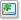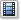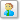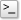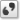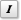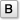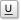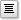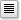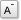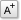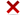Alignement de l'image: Redimensionnement de l'image (en pixel):
Afficher la liste des membres
Pour coloriser votre code, cliquez ici.
Sinon cliquez sur le bouton ci-dessous.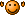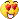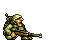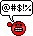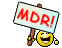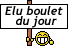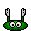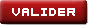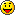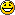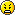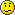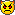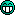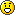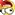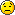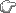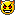Σ π θ ± α β γ δ Δ σ λ
Veuillez donner la réponse en chiffre
Vous devez activer le Javascript dans votre navigateur pour pouvoir valider ce formulaire.

Si vous n'avez pas volontairement désactivé cette fonctionnalité de votre navigateur, il s'agit probablement d'un bug : contactez l'équipe de Planète Casio.

Planète Casio v42 © créé par Neuronix et Muelsaco 2004 - 2020 | Il y a 54 connectés | Nous contacter | Qui sommes-nous ? | Licences et remerciements

Planète Casio est un site communautaire non affilié à Casio. Toute reproduction de Planète Casio, même partielle, est interdite.
Les programmes et autres publications présentes sur Planète Casio restent la propriété de leurs auteurs et peuvent être soumis à des licences ou copyrights.
CASIO est une marque déposée par CASIO Computer Co., Ltd